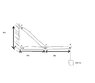# Statics: Mechanical Equilibrium

teknodude
I need some help in this problem to see if my game plan is right. My answer doesn't make sense, well to me it doesn't.

A weight of 200 lb hangs from a frame of negligible weight, as shown. If the frame is in equilibrium, determine the loads acting on the frame at A and D. Use the fact that the frame acts as a three-foce element to check your answer.

-they are all pin connections
-theres a 45 45 90 degree triangle there.

First off i drew a FBD for just DB, and found that it is a two force element, so the line of action passes through the force points of application.

Then when i draw a FBD for ABC the forces at B, it is just a force pointing at a 45 degree angle. Then there's a force Ay and Ax at A and the 200 lb force acting downward at C.

the sum of the forces in the x direction

-Ax -B cos45 = 0

The sum of the force in the y direction

Ay + B sin45 - 200 lb = 0

The sum of the moments in the z direction at point A

-(6ft)(200lb) + (3ft)(Bsin45) = 0

#### Attachments

•untitled.JPG
11.4 KB · Views: 690
Last edited:

teknodude
ok i think I'm using the idea of a 2-force element wrong.

The diagram that i have there needs to be zoomed in a bit, doesn't seem to show up right cause some browsers resize it.
If you need a better diagram then i'll try to scan it this time instead of using paint.

Cyrus
Member DB is a two force member, that makes the analysis simple.

teknodude
At A, for the force i get

0 for the x component
200 lb for the y component

anyone else get these values at point A?

Cyrus
no, Fax=400lb, Fay= 200N down

stellar12533
I got it. I was making this problem more harder than it seemed